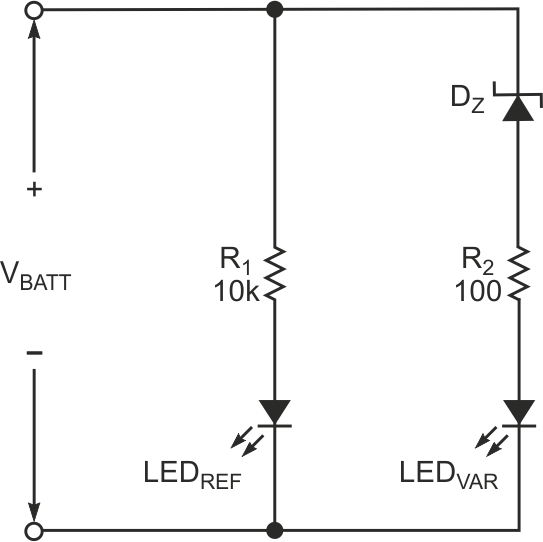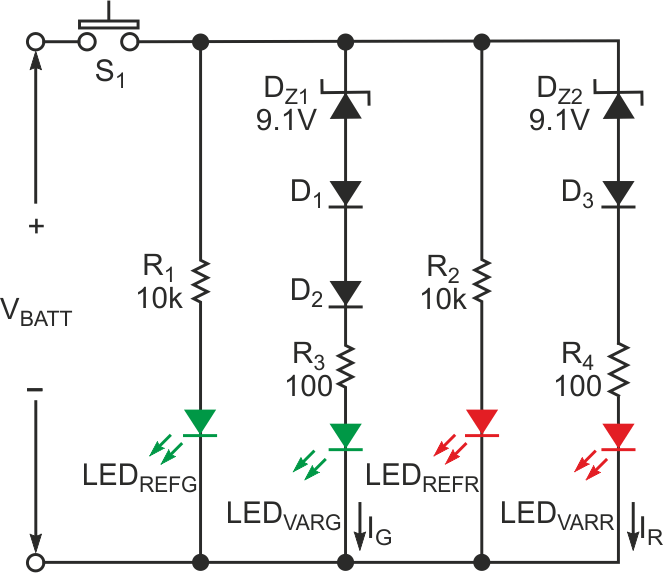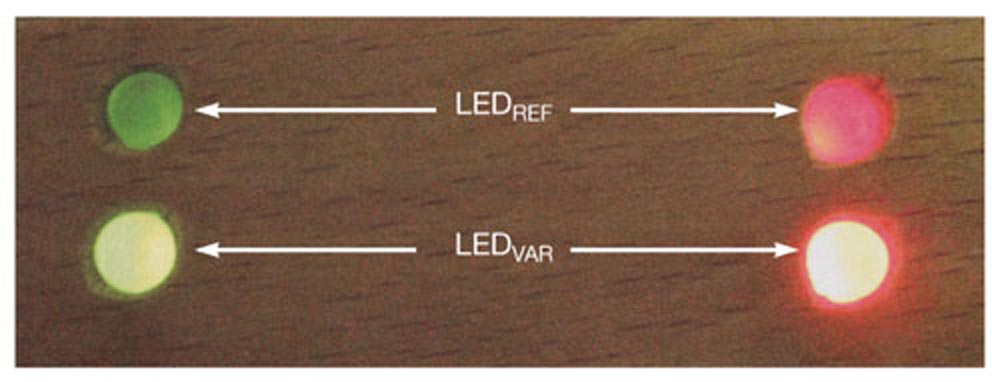# Simple battery-status indicator uses two LEDs

Abhijeet Deshpande

EDN

Properly maintained rechargeable batteries can provide good service and long life. Maintenance involves regular monitoring of battery voltage. The circuit in Figure 1 works in most rechargeable batteries. It comprises a reference LED, LEDREF, which operates at a constant current of 1 mA and provides reference light of constant intensity regardless of battery voltage. It accomplishes this task by connecting resistor R1 in series with the diode. Therefore, even if the battery voltage changes from a charged state to a discharged state, the change in current is only 10%. Thus, the intensity of LEDREF remains constant for a battery state from a fully charged state to a fully discharged state.

The light output of the variable LED changes with respect to changes in battery voltage. The side-by-side-mounted LEDs let you easily compare light intensities and, thus, battery status. Using diffused LEDs as crystal-clear LEDs can damage your eyes. Instead, mount the LEDs with sufficient optical isolation so that the light from one LED does not affect the intensity of the other LEDs.Figure 1. This circuit works in most rechargeable batteries. It comprises a reference LED, LEDREF, which operates at a constant current of 1 mA and provides reference light of constant intensity regardless of battery voltage.

The variable LED operates from 10 mA to less than 1 mA as the battery voltage changes from fully charged to fully discharged. Zener diode DZ in series with resistor R2 causes the current to change with battery voltage. The sum of the zener voltage and the drop across the LED should be slightly less than the lowest battery voltage. This voltage appears across R2. As the battery voltage varies, it produces a large variation of current in R2. If the voltage is approximately 1 V, then 10 mA will flow through LEDVAR, which is much brighter than LEDREF. If the voltage is less than 0.1 V, then the light intensity of LEDVAR will be less than LEDREF, indicating that the battery has discharged.

Immediately after the battery has charged, the battery voltage is more than 13 V. The circuit can withstand this voltage because it has a 10-mA margin. If the LEDs are bright, quickly release pushbutton switch S1 to avoid damage to the LEDs (Figure 2).Figure 2. This circuit can withstand 13 V because it has a 10-mA margin. If the LEDs are bright, quickly release pushbutton switch S1.

The figure uses a 12 V lead-acid battery indicator as an example, but you can extend the design to accommodate other types of chargeable batteries. You can also use it for voltage monitoring. It uses two green LEDs to indicate whether the battery has charged above 60%. A set of red LEDs indicates whether the battery charge drops below 20%. LEDREFG and LEDREFR feed through 10-kΩ resistors R1 and R2. For the variable-intensity LEDs, a zener diode works in series with 100 Ω resistors R3 and R4. Diodes D1, D2, and D3 provide the required clamping voltages. Table 1 shows how LED intensity indicates battery charge.

Table 1. LED intensity
 Light output of LEDVARG Light output of LEDVARR Battery status (%) Much brighter than LEDREFG Much brighter than LEDREFR 70 to 100 Equally as bright as LEDREFG Much brighter than LEDREFR 60 Off Brighter than LEDREFR 50 to 30 Off Equally as bright as LEDREFR 20 Off Off 0 to 10

The following equation calculates the variable intensity for the green LED:

VBATT = IG × 100 + VD1 + VD2 + VLEDG + VDZ1.

For a green-LED current of 1 mA,

VBATT = 10−3 × 100 + 0.6 + 0.6 + 1.85 + 9.1 = 12.25 V.

The selected LEDs have a drop of 1.85 V at 1 mA.

If the LED has different characteristics, then you must recalculate the resistor values. At this voltage, the LEDs have the same intensity, and the battery is 60% charged. See Reference 1 for lead-acid-battery voltages.

The following equation calculates the variable intensity for the red LED:

VBATT = IR × 100 + VD3 + VLEDR + VZD2.

For a green-LED current of 1 mA,

VBATT = 10−3 × 100 + 0.6 + 1.85 + 9.1 = 11.65 V.Figure 3. Both variable-intensity LEDs are brighter than the reference LEDs, indicating that the battery is 100% charged.

At this voltage, both red LEDs have equal intensities, and the battery is 20% charged. LEDVARG is off. Figure 3 shows that both variable-intensity LEDs are brighter than the reference LEDs, indicating that the battery is 100% charged.

## Reference

EDNYou may have to register before you can post comments and get full access to forum.
 User Name Remember Me? Password